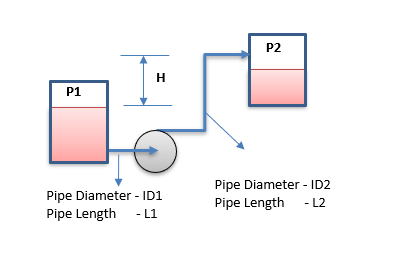# Pump Operation Series & Parallel

This web application determine change in pump head vs flow curve when two similar pumps are operating in either series or parallel configuration.

Pump Curve

Pump SystemPump in Series

In series arrangement, each pump handles same flow rate, but the total head produced by the combination of pumps will be additive. Since each pump generates a head H corresponding to a flow Q, when connected in series, the total head developed is Ht = H1 + H2, where H1, H2 are the heads developed by the pump in series at the common flow rate Q.

Pump in Parallel

With pumps in parallel, the flow rates are additive with a common head. The flowrate Qt is split between the inlet into Q1 and Q2. Each pump develops the same head H at the corresponding capacity. Thus, the first pump at capacity Q1 develops the same head H as the second pump at capacity Q2.

Pump System

m
mm

Suction Side

bara
mm
m

Discharge Side

bara
mm
m